• Category
• >Deep Learning

# Convolutional Neural Network (CNN): Graphical Visualization with Python Code Explanation

• Tanesh Balodi
• Sep 06, 2019
• Updated on: Feb 11, 2021Convolutional neural networks are neural networks that are mostly used in image classification, object detection, face recognition, self-driving cars, robotics, neural style transfer, video recognition, recommendation systems, etc.

CNN classification takes any input image and finds a pattern in the image, processes it, and classifies it in various categories which are like Car, Animal, Bottle, etc. CNN is also used in unsupervised learning for clustering images by similarity. It is a very interesting and complex algorithm, which is driving the future of technology.

## Topics Covered

1. What is Convolutional Neural Network (CNN)?

2. Convolutional Layer and Max-pooling Layer

3. Activation Functions

4. Fully Connected Network (FCN)

5. Conclusion

## What is Convolutional Neural Network (CNN)?

“Convolution neural networks” indicates that these are simply neural networks with some mathematical operation (generally matrix multiplication) in between their layers called convolution.

It was proposed by Yann LeCun in 1998. It's one of the most popular uses in Image Classification. Convolution neural network can broadly be classified into these steps :

1. Input layer

2. Convolutional layer

3. Output layers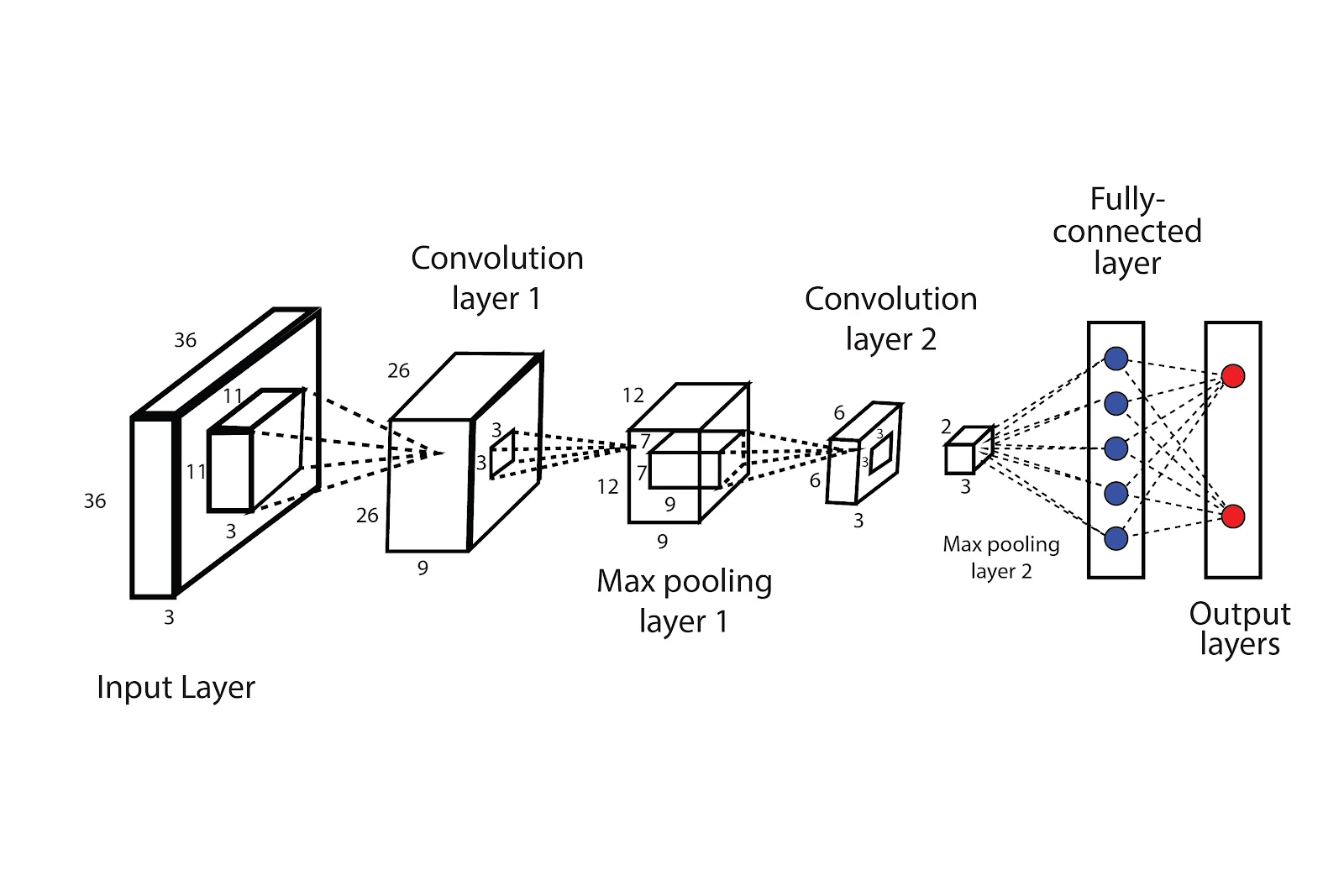The architecture of Convolutional Neural Networks(CNN)

Input layers are connected with convolutional layers that perform many tasks such as padding, striding, the functioning of kernels, and so many performances of this layer, this layer is considered as a building block of convolutional neural networks.

(Speaking of convolutional neural networks, you can also check out our blog on Introduction to Common Architectures in Convolution Neural Networks)

We will be discussing it’s functioning in detail and how the fully connected networks work.

## Convolutional Layer

The convolutional layer’s main objective is to extract features from images and learn all the features of the image which would help in object detection techniques.

As we know, the input layer will contain some pixel values with some weight and height, our kernels or filters will convolve around the input layer and give results which will retrieve all the features with fewer dimensions. Let’s see how kernels work;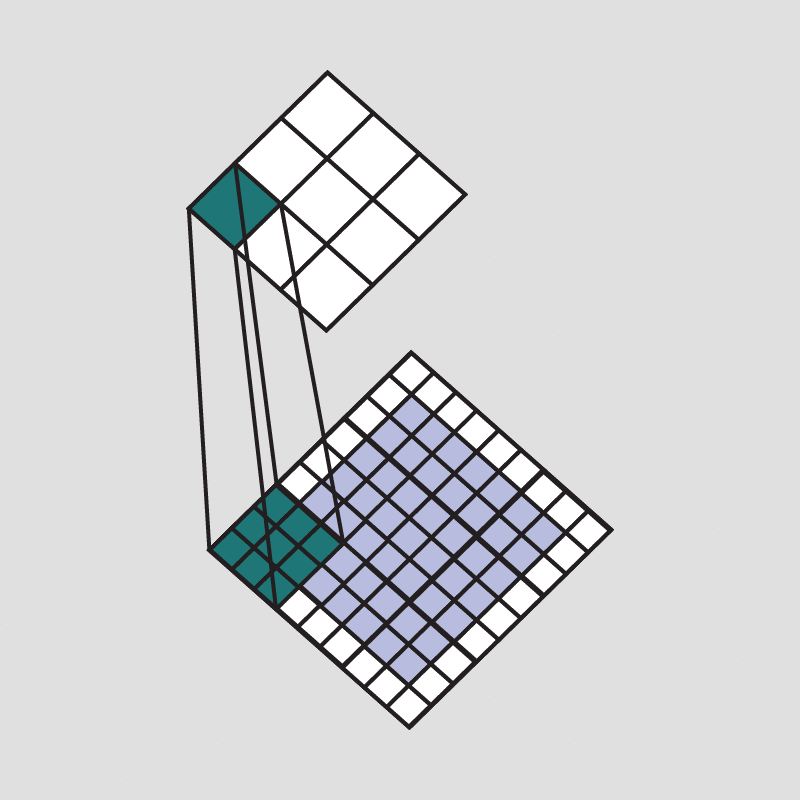Formation and arrangement of Convolutional Kernels

With the help of this very informative visualization about kernels, we can see how the kernels work and how padding is done.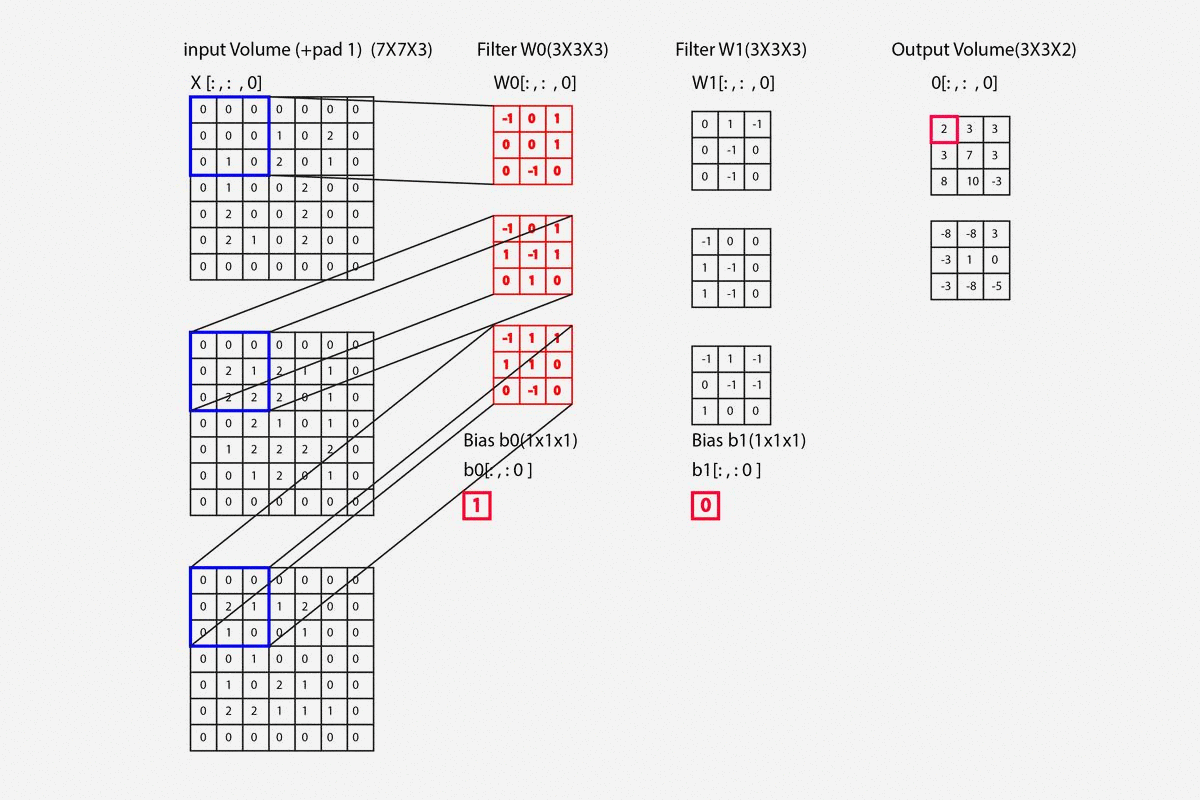Matrix visualization in CNN

We can see padding in our input volume, we need to do padding in order to make our kernels fit the input matrices. Sometimes we do zero paddings, i.e. adding one row or column to each side of zero matrices or we can cut out the part, which is not fitting in the input image, also known as valid padding.

Let’s see how we reduce parameters with negligible loss, we use techniques like Max-pooling and average pooling.

## Max pooling or Average pooling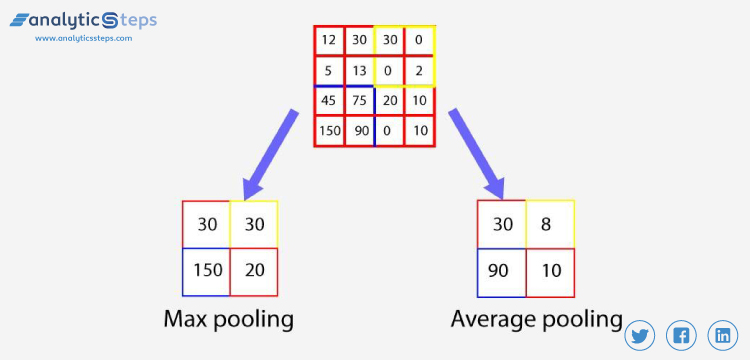Matrix formation using Max-pooling and average pooling

Max pooling or average pooling reduces the parameters to increase the computation of our convolutional architecture. Here, 2*2 filters and 2 strides are taken  (which we usually use).

By name, we can easily assume that max-pooling extracts the maximum value from the filter and average pooling takes out the average from the filter. We perform pooling to reduce dimensionality. We have to add padding only if necessary.

The more convolutional layer can be added to our model until conditions are satisfied.

## Activation Functions in CNN

An activation function is added to our network anywhere in between two convolutional layers or at the end of the network. So you must be wondering what exactly an activation function does, let me clear it in simple words for you.

It helps in making the decision about which information should fire forward and which not by making decisions at the end of any network. In broadly, there are both linear as well as non-linear activation functions, both performing linear and non-linear transformations but non-linear activation functions are a lot helpful and therefore widely used in neural networks as well as deep learning networks.

The four most famous activation functions to add non-linearity to the network are described below.

### 1. Sigmoid Activation Function

The equation for the sigmoid function is

f(x)    =      1/(1+e-X )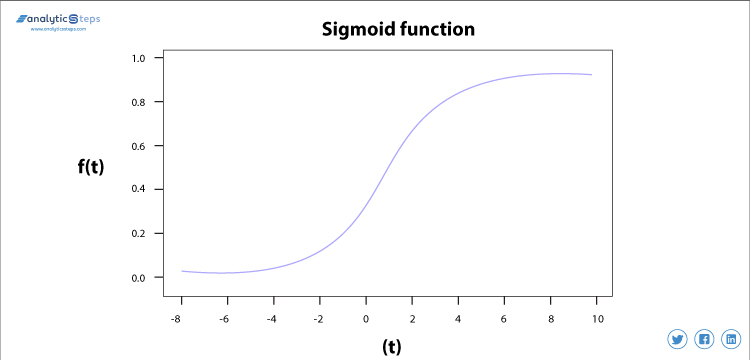Sigmoid Activation function

The sigmoid activation function is used mostly as it does its task with great efficiency, it basically is a probabilistic approach towards decision making and ranges in between 0 to 1, so when we have to make a decision or to predict an output we use this activation function because of the range is the minimum, therefore, the prediction would be more accurate.

### 2. Hyperbolic Tangent Activation Function(Tanh)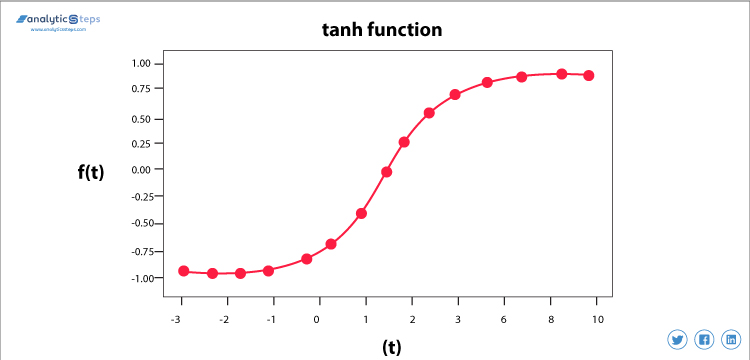Tanh Activation function

This activation function is slightly better than the sigmoid function, like the sigmoid function it is also used to predict or to differentiate between two classes but it maps the negative input into negative quantity only and ranges in between -1 to 1.

### 3. ReLU (Rectified Linear unit) Activation function

Rectified linear unit or ReLU is the most widely used activation function right now which ranges from 0 to infinity, all the negative values are converted into zero, and this conversion rate is so fast that neither it can map nor fit into data properly which creates a problem, but where there is a problem there is a solution.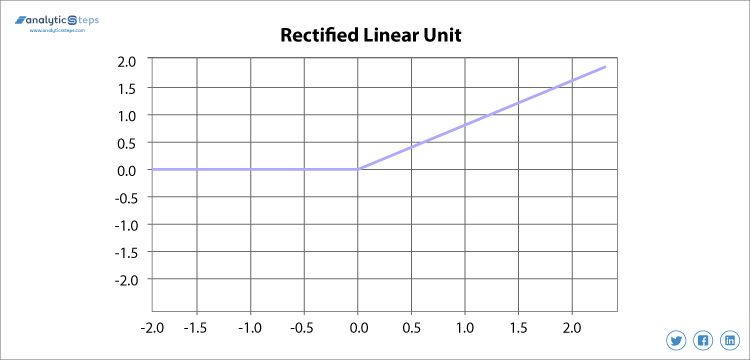Rectified Linear Unit activation function

We use Leaky ReLU function instead of ReLU to avoid this unfitting, in Leaky ReLU range is expanded which enhances the performance.

### 4. Softmax Activation Function

Softmax is used mainly at the last layer i.e output layer for decision making the same as sigmoid activation works, the softmax basically gives value to the input variable according to their weight, and the sum of these weights is eventually one.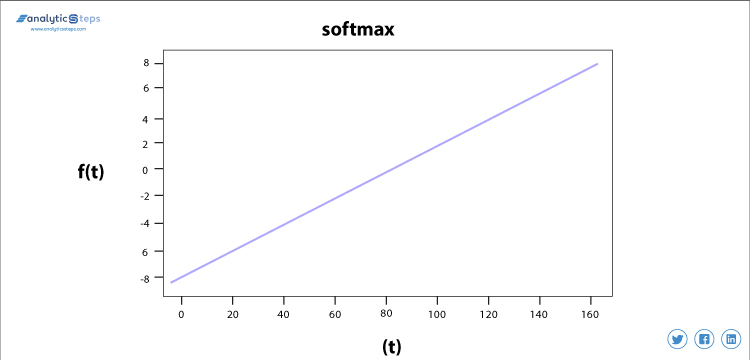Softmax activation function

For Binary classification, both sigmoid, as well as softmax, are equally approachable but in the case of multi-class classification problems we generally use softmax and cross-entropy along with it.

## Fully Connected Network (FCN)View to Fully Connected Network (FCN)

In our last layer which is a fully connected network, we will be sending our flatten data to a fully connected network, we basically transform our data to make classes that we require to get from our network as an output.

Majorly there are 7 types of Activation Functions in Neural Network that are used in neural networks as well as in other machine learning algorithms.

## Python code for the Convolutional Neural Network

### Step 1

Importing all necessary libraries(mainly from Keras)

``````import numpy as np

import matplotlib.pyplot as plt

from sklearn.model_selection import train_test_split

import keras

from keras.models import Sequential

from keras.layers import Conv2D, MaxPool2D, Dense, Flatten, Activation

from keras.utils import np_util``````

Importing sequential model, activation, dense, flatten, max-pooling libraries.

### Step 2

Importing dataset. If you want to use the same dataset you can download.

``````dataset = read_csv(r'Fashion mnist')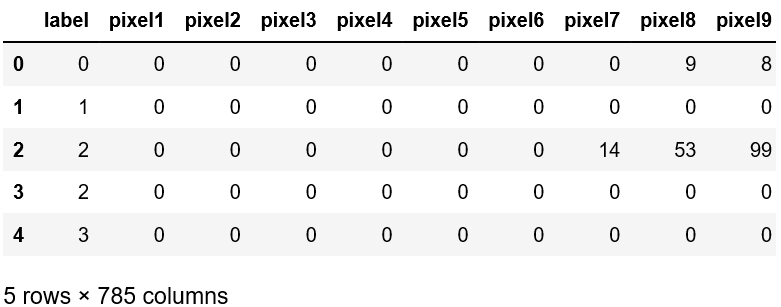### Step 3

``dataset.values``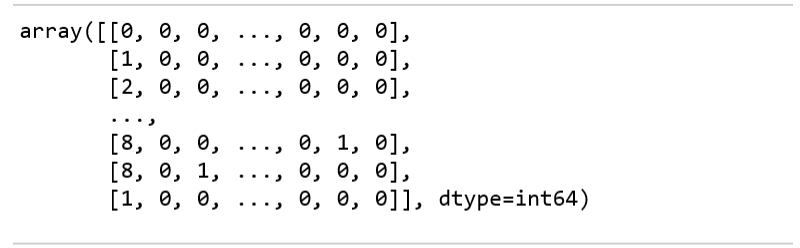Visualizing Data Type in Array Form

Visualizing our dataset and splitting into training and testing. Here, np.utils converts a class integer to the binary class matrix for use with categorical cross-entropy.

### Step 4

``X_train.shape, X_test.shape, y_train.shape, y_test.shape``

((8000, 784), (2000, 784), (8000, 10), (2000, 10))

``````X_train, X_test = X_train.reshape((-1,28,28,1)), X_test.reshape((-1,28,28,1))

X_train.shape, X_test.shape, y_train.shape, y_test.shape``````

((8000, 28, 28, 1), (2000, 28, 28, 1), (8000, 10), (2000, 10))

Reshaping our x_train and x_test for use in conv2D. And we can observe the change in the shape of our data.

### Step 5

``````model = Sequential()

# Conv1

# Conv2

model.summary()

``````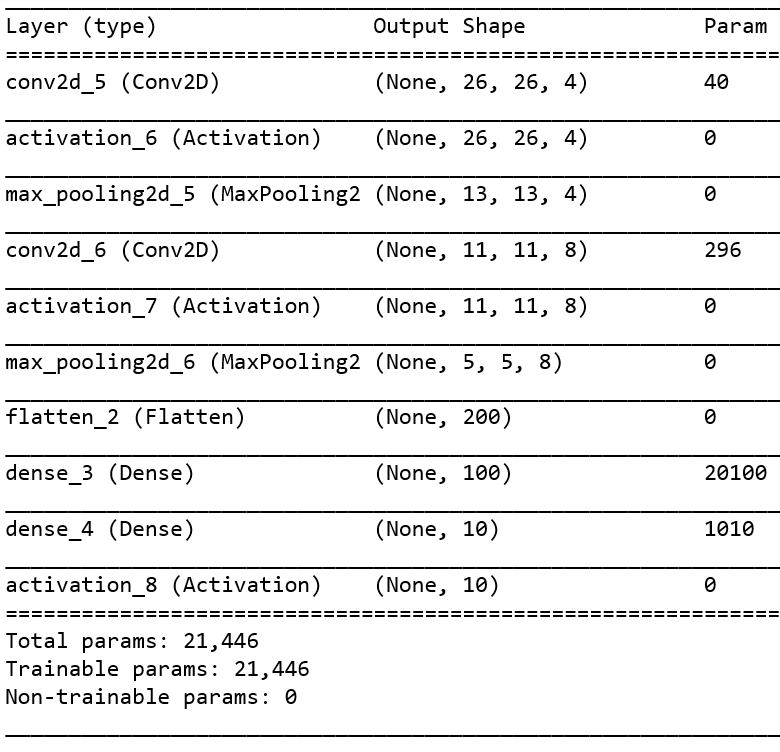Implementing CNN Structure

This is the main structural part of CNN, where CNN is implemented, we have taken two convolutional layers and we can see we have added different activation functions like ReLU, sigmoid, and softmax function. Our structure goes in accordance with what we have already discussed above.

### Step 6

``````model.compile(loss='categorical_crossentropy',

metrics=['accuracy'])``````

To compute loss, we use categorical cross-entropy, for more functionality of Keras, you can visit the documentation of Keras from keras.org.

### Step 7

``````hist = model.fit(X_train, y_train,

shuffle=True,

batch_size=128,

epochs=30,

validation_data=(X_test, y_test)

)

``````

Fitted our training data to our model and took the batch size as 128, which will take 128 values at once till total parameters are satisfied. Here epochs mean the number of times it will be processed.

### Step 8

``````plt.figure(0)

plt.title("Loss")

plt.plot(hist.history['loss'], 'r', label='Training')

plt.plot(hist.history['val_loss'], 'b', label='Testing')

plt.legend()

plt.show()``````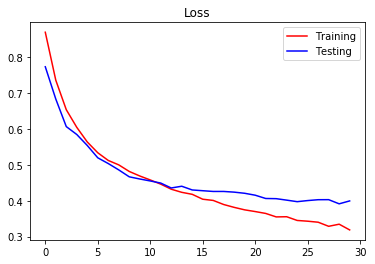Computing Loss Result on Training And Test Results

The plot for loss between the training set and testing set.

``````plt.figure(1)

plt.title("Accuracy")

plt.plot(hist.history['acc'], 'r', label='Training')

plt.plot(hist.history['val_acc'], 'b', label='Testing')

plt.legend()

plt.show()``````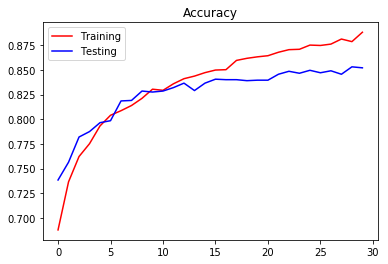Computing Accuracy on Training And Test Results

The plot for accuracy on the training set and test set has been visualized with the help of the matplotlib. We can easily determine the difference between the accuracy of training and the test set by a simple analysis of the graph.

## Conclusion

CNN is the best artificial neural network technique, it is used for modeling images but it is not limited to just modeling of the image but out of many of its applications, there is some real-time object detection problem that can be solved with the help of this architecture.

There are many improvised versions based on CNN architecture like AlexNet, VGG, YOLO, and many more that have advanced applications on object detection.

•#### servicetechlaayounetv

Aug 14, 2022

code source not works

•#### carlosgiorgio

Oct 19, 2022

for me too, code source not works

•